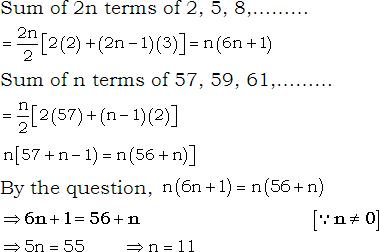Courses

# JEE(MAIN) Mathematics Mock Test - 1

## 30 Questions MCQ Test JEE Main Mock Test Series 2020 & Previous Year Papers | JEE(MAIN) Mathematics Mock Test - 1

Description
This mock test of JEE(MAIN) Mathematics Mock Test - 1 for JEE helps you for every JEE entrance exam. This contains 30 Multiple Choice Questions for JEE JEE(MAIN) Mathematics Mock Test - 1 (mcq) to study with solutions a complete question bank. The solved questions answers in this JEE(MAIN) Mathematics Mock Test - 1 quiz give you a good mix of easy questions and tough questions. JEE students definitely take this JEE(MAIN) Mathematics Mock Test - 1 exercise for a better result in the exam. You can find other JEE(MAIN) Mathematics Mock Test - 1 extra questions, long questions & short questions for JEE on EduRev as well by searching above.
QUESTION: 1

Solution:
QUESTION: 2

Solution:
QUESTION: 3

### In the following question, a Statement of Assertion (A) is given followed by a corresponding Reason (R) just below it. Read the Statements carefully and mark the correct answer- Assertion(A) :The inverse of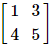does not exist. Reason(R) :The matrix is non singular.

Solution:
QUESTION: 4

If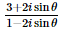sin θ is real, then θ =

Solution:
QUESTION: 5

The length of the tangent from (0,0) to the circle 2x2 + 2y2 + x - y + 5 = 0 is

Solution:
QUESTION: 6

If the line 3x-4y=λ touches the circle x2+y2-4x-8y-5=0, λ  can have the values

Solution:
QUESTION: 7

If sin y = x sin (a + y), then (dy/dx) =

Solution:
QUESTION: 8

The differential equation which represents the family of plane curves y=exp. (cx) is

Solution:

y = ecx
dy/dx = c. ecx
y' = cy

QUESTION: 9
If A, B, C are represented by 3 + 4i, 5 - 2i, -1 + 16i respectively, then A, B, C are
Solution:
QUESTION: 10

The fundamental period of the function f(x) = 2 cos 1/3(x - π) is

Solution:
QUESTION: 11
Which of the following is not a statement ?
Solution:
QUESTION: 12
In the following question, a Statement-1 is given followed by a corresponding Statement-2 just below it. Read the statements carefully and mark the correct answer-
Consider the planes 3x – 6y – 2z = 15 and 2x + y – 2z = 5.
Statement-1:
The parametric equations of the line of intersection of the given planes are
x = 3 + 14t, y = 1 + 2t, z = 15t.
Statement-2:
The vector 14î+2ĵ+15k̂ is parallel to the line of intersection of given planes
Solution:
QUESTION: 13

In the following question, a Statement-1 is given followed by a corresponding Statement-2 just below it. Read the statements carefully and mark the correct answer-
Tangents are drawn from the point (17,7) to the circle x2+y2=169.
Statement-1:
The tangents are mutually perpendicular.
Statement-2:
The locus of the points from which mutually perpendicular tangents can be drawn to the given circle is x2+y2=338.

Solution: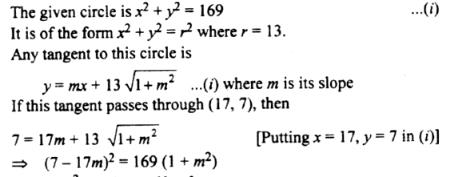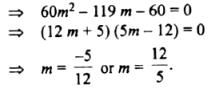Clearly, m1m2 = - 1.
Hence, the two tangents arc mutually perpendicular.
Statement 1 is true.
Now, the locus of the point of intersection of two mutually perpendicular tangents to the circle x2 + y2 = r2 is the director circle i.e. the circle x2 +y2 = 2r2
For the given circle r = 13. ..
Its director circle is x2 + y2 = 338.
Hence, statement 2 is true and  a cogect explanation of statement as the point (17, 7) lies on the director circle of the circle (i).

QUESTION: 14

The value of a for which the system of equations
a3x+(a+1)3y+(a+2)3z = 0
ax+(a+1)y+(a+2)z = 0
x+y+z = 0
has a non-zero solution, is

Solution:

The system of equation has a non-zero solution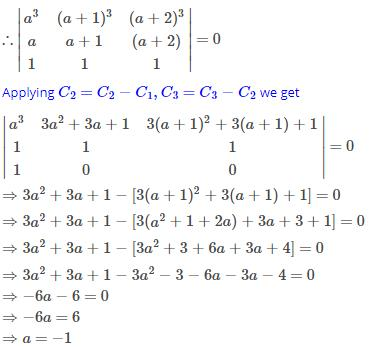QUESTION: 15

The pole of the line 2x + 3y − 4 = 0 with respect to the parabola y2 = 4 x is

Solution:
QUESTION: 16

If nC12=nC8, then n=

Solution:QUESTION: 17

The chance of getting a doublet with 2 dice is

Solution:

Total outcomes = 36
Doublet are 6 (1,1),(2,2),(3,3),(4,4),(5,5),(6,6)

Probability of getting doublet = 6/36
= 1/6

QUESTION: 18

If cov. (x, y) = 0, then ρ(x, y) equals

Solution:

Covariance is a quantitative measure of the extent to which the deviation of one variable from its mean matches the deviation of the other from its mean. It is a mathematical relationship that is defined as:

Cov(X,Y) = E[(X − E[X])(Y − E[Y])]

Correlation between two random variables, ρ(X,Y) is the covariance of the two variables normalized by the variance of each variable. This normalization cancels the units out and normalizes the measure so that it is always in the range [0, 1]:QUESTION: 19

The two opposite vertices of a square on xy-plane are A(-1,1) and B(5,3), the equation of other diagonal (not passing through A and B) is

Solution:

Given: Here,AB is the diagonal of square.
The vertices of a square A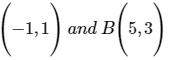Let the mid−point of AB be EThen coordinates of E are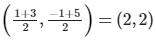Therefore equation of other diagonal is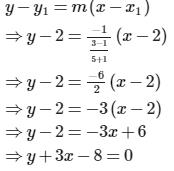QUESTION: 20

If the normal to the curve y=f(x) at the point (3,4) makes an angle 3π/4 with the positive x-axis, then f'(3)

Solution:

Given y = f(x)

differentiating w.r.t x

y' = f'(x) which is the slope of the tangent

Hence the slope of the normal is - 1/f'(x) = 3pi/4 = -1

therefore f'(x) = 1

Hence f'(3) = 1

QUESTION: 21

The intersection of the spheres x2 + y2 + z2 + 7x - 2y - z = 13 and x2 + y2 + z2 - 3x + 3y + 4z = 8 is the same as the intersection of one of the sphere and the plane

Solution:

Required plane is S1 – S2 = 0 where
S1 = x2 + y2 + z2 + 7x – 2y – z – 13 = 0
and S2 = x2 + y2 + z2 – 3x + 3y + 4z – 8 = 0
⇒ 2x – y – z = 1.

QUESTION: 22

If θ and φ are + ve actue angles and tan(θ+φ)=1,(θ-φ)=1/√3,φ equlas

Solution:
QUESTION: 23

The sine of the angle between the vectors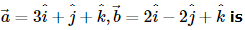Solution:
QUESTION: 24

Let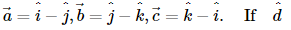is a unit vector such that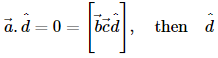equals

Solution:
QUESTION: 25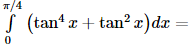Solution:
QUESTION: 26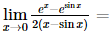Solution: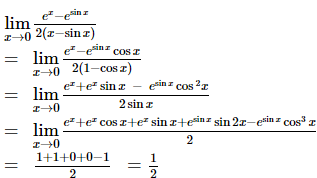QUESTION: 27

In the following question, a Statement of Assertion (A) is given followed by a corresponding Reason (R) just below it. Read the Statements carefully and mark the correct answer-
Assertion(A): The system of the equations
2x + 4y + 1 = 0
4x + 8y + 3 = 0 has no solution.
Reason(R): The system of equations
a1x + b1y + c1 = 0
a2x + b2y + c2 = 0 has no solution , if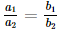Solution:
QUESTION: 28

If A is a square matrix of order 3 and entries of A are positive integers, then |A| is

Solution: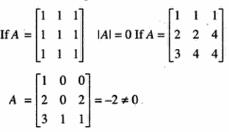Also, |A| is Negative. Hence, |A| can be arbitrary integer.

QUESTION: 29

For all real x, the minimum value of [(1-x+x2)/(1+x+x2)] is

Solution:
QUESTION: 30

If the sum of the first 2n terms of the A.P. 2, 5, 8, ..., is equal to the sum of the first nn terms of A.P. 57, 59, 61, ..., then nn equals

Solution: